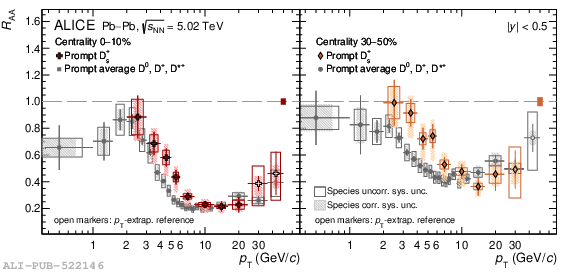# Figure 4

 Nuclear modification factor $\RAA$ of prompt $\Ds$ mesons in the 0--10\% (left panel) and 30--50\% (right panel) centrality intervals in Pb--Pb collisions at $\sqrtsNN=5.02 \TeV$ compared with the one of prompt non-strange D mesons (average of $\Dzero$, $\Dplus$, and $\Dstar$) . The empty (filled) boxes represent the species uncorrelated (correlated) systematic uncertainties. The normalisation uncertainty is represented by a filled box at $\RAA=1$.